# The ring of entire functions

Rings made a bad first impression on me. I couldn’t remember the definitions of all the different kinds of rings, much less have an intuition for what was important about each one. As I recall, all the examples of rings in our course were variations on the integers, often artificial variations.

## Entire functions

I’m more interested in analysis than algebra, so my curiosity was piqued when I ran across an appendix on entire functions in the back of an algebra book . This appendix opens with the statement

The ring E of entire functions is a most peculiar ring.

It’s interesting that something so natural from the perspective of analysis is considered peculiar from the perspective of algebra.

An entire function is a function of a complex variable that is analytic in the entire complex plane. Entire functions are functions that have a Taylor series with infinite radius of convergence.

## Rings

A ring is a set of things with addition and multiplication operations, and these operations interact as you’d expect via distributive rules. You can add, subtract, and multiply, but not divide: addition is invertible but multiplication is not in general. Clearly the sum or product of entire functions is an entire function. But the reciprocal of an entire function is not an entire function because the reciprocal has poles where the original function has zeros.

So why is the ring of analytic functions peculiar to an algebraist? Osborne speaks of “the Jekyll-Hyde nature of E,” meaning that E is easy to work with in some ways but not in others. If Santa were an algebraist, he would say E is both naughty and nice.

## Nice properties

On the nice side, E is an integral domain. That is, if f and g are entire functions and fg = 0, then either f = 0 or g = 0.

If we were looking at functions that were merely continuous, it would be possible for f to be zero in some places and g to be zero in the rest, so that the product fg is zero everywhere.

But analytic functions are much less flexible than continuous functions. If an analytic function is zero on a set of points with a limit point, it’s zero everywhere. If every point in the complex plane is either a zero of f or a zero of g, one of these sets of zeros must have a limit point.

Another nice property of E is that it is a Bezóut domain. This means that if f and g are entire functions with no shared zeros, there exist entire functions λ and μ such that

λf + μg = 1.

This is definition is analogous to (and motivated by) Bezóut’s theorem in number theory which says that if a and b are relatively prime integers, then there are integers m and n such that

ma + nb = 1.

## Naughty properties

The naughty properties of E take longer to describe and involve dimension. “Nice” rings have small Krull dimension. For example Artinian rings have Krull dimension 0, and the ring of polynomials in n complex variables has dimension n. But the Krull dimension of the ring of entire functions is infinite. In fact it’s “very infinite” in the sense that it is at leastwhere ℵ1 is bigger than ℵ0, the next cardinal after ℵ0 if you accept the continuum hypothesis. So the Krull dimension of E is larger than the cardinality of the complex numbers.

## Wittgenstein’s ruler

Nassim Taleb described Wittgenstein’s ruler this way:

Unless you have confidence in the ruler’s reliability, if you use a ruler to measure a table you may also be using the table to measure the ruler. The less you trust the ruler’s reliability, the more information you are getting about the ruler and the less about the table.

An algebraist would say that entire functions are weird, but an analyst could say that on the contrary, ring theory, or at least Krull dimension, is weird.

## Related posts

 Basic Homological Algebra by M. Scott Osborne.

# The awkward middle child of algebra

Abstract algebra is basically the study of groups, rings, and fields. There are more concepts, but these are the big three.

Groups have the least structure and fields have the most structure. Rings are somewhere in the middle.

Groups have just one operation, which is thought of as multiplication by default. If the group operation is commutative, it’s thought of as addition. But in either case, there’s only one operation.

Fields have two operations, addition and multiplication, and the operations have all the basic properties you expect.

Rings also have two operations, addition and multiplication, but they lack some of the familiar properties of fields.

The most awkward thing about ring theory is that there is no universal agreement on the definition of a ring. The disagreement is over whether the definition should require the existence of a multiplicative identity, the analog of the number 1. Most authors include this as part of the definition, but a substantial portion do not.

There are two ways of dealing with this mess. The most common is to require rings to have an identity, but continually remind readers of this convention. And so you’ll see things like “Let R be a ring (with identity).”

The other less common solution is to give custody of the term “ring” to those who require an identity and use the term “rng” for the thing that’s just like a ring except it doesn’t necessarily have an identity.

Another awkward, or at least curious, feature of ring theory is that you almost never speak of subrings. Subgroups are important in group theory, and subfields are important in field theory . But you almost never hear the word “subring.”

The ring theory counterpart to subgroups in group theory is the ideal. The name comes from Earnst Kummer thinking of these objects as ideal numbers, numbers added to an algebraic system to fill in some deficiency.

Given a ring R, a subset I of R is an ideal if it is closed under addition and under multiplication by any element of R. Note the asymmetry between addition and multiplication. You can add two elements of I and stay in I. And you can multiply an element in I, not just by another element of I, but by any element in R, and stay in I.

A quick example will help. Let R be the integers and let I be all multiples of 7. The sum of two multiples of 7 is another multiple of 7, And if you multiply a multiple of 7 by any integer you get another multiple of 7. So multiples of 7 form an ideal in the integers.

The disagreement over whether to require identities and the lack of interest in subrings are related. If you don’t require a ring to have an identity element, then you don’t require subrings to have an identity element, and so every ideal is a subring. But if you do require rings to have an identity, the only ideal of R that is a subring is R itself.

A final awkward thing about ring theory is that there are so many kinds of rings: integral domains, principle ideal domains, Noetherian rings, Artinian rings, etc. It seems that “ring” itself is too generic to be useful except as a pedagogical device to organize what more specific kinds of rings have in common.

In this sense ring theory is analogous to “absolute geometry,” the study of the common features of Euclidean and Non-Euclidean geometry. In practice one cares about more specific geometries, but there’s some value in cataloging theorems that various geometries share.

## Related posts

 The concept of a subfield is more common than the term “subfield.” In field theory, it’s more common to say that K is an extension field of F than to say that F is a subfield of K, though the two statements are equivalent.

# Partial functions and total functions

I was thinking about writing a post about entire functions and it occurred to me that I should say something about how entire functions are unrelated to total functions. But then I realized they’re not unrelated. I intend to say something about entire functions in a future post.

Update: See The ring of entire functions.

## Partial functions

A total function is a function defined for every element of its domain. This terminology is more common in computer science than in math. A lot of what we call functions in software are actually partial functions because they’re not defined everywhere. For example, the square root function sqrt in C takes a argument of type double and returns a value of type double, but it’s not defined for all inputs of type double, only those inputs that are not negative.

Mathematically speaking it makes no sense to refer to a function that is not defined on every point of its domain. A function’s domain is by definition the set of points where it is defined, so a total function is just a function, and a partial function is not even a function.

And yet it is convenient to refer informally to functions that are undefined for some inputs. This is common as dirt in software development, but it occurs in math too.

## Type checking

The distinction between total functions and partial functions is more subtle than it may seem.

For example, it would be more precise to say that the sqrt function is defined on non-negative numbers of type double. There’s no non-negative floating point type in C, but in principle we could introduce one. Sounds like a good idea. Why hasn’t C done this?

It’s obvious when functions are defined on (some) arguments of type double and return (some) arguments of type double.  It’s not so obvious that functions are defined on more restricted types or return more restrictive types. For example, suppose you want to apply sqrt to a function that you wrote. If your function takes in a double and squares it, then this is fine. It may ostensibly return a double but in fact it returns a non-negative double. But if your function cubes its input then the output may not be compatible with the input to sqrt.

The domain of the square root function is simple, but what about functions that are undefined at more complicated sets. For example, the gamma function is undefined at 0 and at negative integers. The gamma function is undefined at -4, for instance, but it’s defined at -4 + ε for any tiny ε. Imagine how hard it might be to verify that a function returns -4 + ε but not -4.

Or suppose you have a function that computes the real part of complex numbers for which the Riemann zeta function is zero. If you could prove that the program always returns either 1/2 or a negative even integer, you would earn fame and fortune. This is an extreme example, but it is true that determining the precise domain or range of a function can require proving theorems that are difficult if not impossible.

## Dependent type theory

We’re at a fork in the road. We either give up on more restrictive types and live with functions that may not be defined for every element in their domain, or we implement far more sophisticated types and type checking. In other words, we get into dependent type theory.

If we choose the fork leading to dependent types, there are a lot of new costs and new benefits. The benefits are more obvious than the costs. The benefits include, for example, having a compiler reject code that could pass a negative value into sqrt. But this comes at the expense of making it far more difficult to write compilers. It also restricts the range of programs that can be written or increases the skill requirement of those who can write the needed programs.

## Mathematical formalism

Mathematicians informally speak of functions not being defined everywhere in their domain even though it would be more accurate to say that the domain excludes certain points. This kind of talk is fine among friends where there’s an implicit understanding of what detail is being left out and the trust that the details will be made more explicit when necessary. In a more hostile environment, like Twitter, pedants will gleefully pounce on such lapses in rigor.

You can make partial functions rigorous by defining them to be relations rather than functions. A relation between sets A and B is a subset of their Cartesian product A × B. A function is a relation such that for every a in A there exists a unique pair (a, b) in the relation. A partial function is a relation in which for every a in A there is at most one pair (a, b) in the relation.

A “multi-valued function” is strictly speaking an oxymoron, but more formally it is a also relation, not a function. As with partial functions, the terminology “multi-valued function” is informal. Typically there is a way to formalize multi-valued functions so that they are actual (single-valued) functions with a different codomain, but one may wish to avoid this extra formality when it is not necessary.

# Exact sequences

A couple days ago, near the end of a post, I mentioned exact sequences. This term does not mean what you might reasonably think it means. It doesn’t mean exact in the sense of not being approximate.

It means that the stuff that comes out of one step is exactly the stuff that gets set to 0 in the next step. That is, the image of each function is exactly the kernel of the next function in the sequence .

For example, let f be gradient and let g be curl. Then the following sequence is exactif A is the set of smooth functions from ℝ³ to ℝ, and B and C are set of smooth functions ℝ³ to ℝ³.

This says that the image of f, vector fields that are the gradient of something, is the kernel of g, vector fields with zero curl. In other lingo, gradient vector fields are irrotational, and all irrotational vector fields are the gradient of some potential function.

To show that

image f = kernel g

we have to show two things:

image f ⊂ kernel g

and

image f ⊃ kernel g

As is often the case, the former is easier than the latter. It’s a common homework problem  to show that the curl of a divergence is zero, i.e.

∇×(∇φ) = 0

It’s not so easy to show that for every vector field F with ∇×F = 0 there exists a potential φ such that F = ∇φ. It’s easier to show that the image of the first function part of the kernel of the next than to show that the kernel of the first is exactly the kernel of the next, because the latter requires proving the existence of something.

## Short exact sequences

A short exact sequence is an exact sequence of the following form. (It’s called short because exact sequences are often longer.)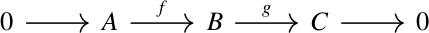There’s no label on the arrow from 0 to A because there’s only one function from 0 to anywhere. There’s also no label on the arrow from C to 0 because there’s only one function that goes from anywhere to 0 .

The zero on the left implies that the function f is one-to-one (injective). It’s image is only one element, so the kernel of f is only one element.

Similarly the zero on the right end implies that the function g is onto (surjective). The kernel of the last arrow is everything in C, so the image of g has to be everything in C.

For example, let B be a group and suppose φ is a homomorphism from B to another group. Let A be the kernel of φ and let f be the inclusion map from the kernel into B. Let g be quotient map taking C = B/A. Then the so-called “first group homomorphism theorem” says that the sequence above is exact.

## Div, grad, curl and all that

The title of this section is an homage to an excellent little book by H. M. Schey.

We said above that the curl of a gradient is zero, and that all vector fields with zero curl are gradients. It’s also true that the divergence of a curl is zero, and that a vector field is has zero divergence if it is the curl of something. That is, for a vector field F,

∇ · (∇ × F) = 0

and if ∇ · G = 0 for a vector field G then there exists a vector field F such that

∇×F = G.

This means we can extend our example above to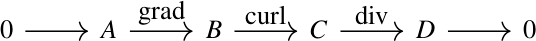if we define A carefully.

The discussion in this section only justifies the labeled arrows. We need to justify the two unlabeled arrows on the ends.

The zero on the left requires the gradient be one-to-one. But in general, the gradient is not one-to-one: functions that differ by a constant have the same gradient. But if we define A to be the set of integrable functions on ℝ³ then the gradient is one-to-one. Requiring the integral of a function over ℝ³ to exist means the functions must eventually approach zero in every direction.

The zero on the right requires every smooth function on ℝ³ to be the divergence of something. That’s easy. Given a function φ from ℝ³ to ℝ, let F be the vector field whose first component at (x, y, z) is the integral of φ from the origin to (x, 0, 0) and whose second and third components are 0. Then the divergence of F equals φ.

## Long(er) exact sequences

The exact sequence in the previous section is longer than a short exact sequence, and longer exact sequences come up in practice. An exact sequence could be infinite in one or both directions. For example, the Mayer–Vietoris sequence, a foundational tool in homology, is infinite on its left and and terminates in 0 on its right end.

## Related posts

 The term “zero” is overloaded here. It could be the integer 0, or the origin of a vector space, or the kernel of a group, etc.

Everything here generalizes to objects and morphisms in a category, in which case the objects are not necessarily sets, and the morphisms are not necessarily functions. Then we don’t talk about images and kernels but about morphisms being monomorphisms and epimorphisms.

 There is a lot of foreshadowing in textbooks. It’s common for an author to include exercises and examples that are important later. This is often intentional, sort of an Easter egg. But sometimes the author is unconsciously pulling from experience.

 This observation is turned into a definition in category theory: a zero object is defined as one that is initial and final. That is, there is only one morphism from it to any other object, and only one morphism from any object to it.

# Data swampsI recently heard the term data swamp, a humorous take on data lakes. I thought about data swamps yesterday when I hiked past the literal swamp in the photo above.

Swamps are a better metaphor than lakes for heterogeneous data collections because a lake is a homogeneous body of water. What makes a swamp a swamp is its heterogeneity.

The term “data lake” may bring up images of clear water, maybe an alpine lake like Lake Tahoe straddling California and Nevada. “Data swamp” makes me think of Louisiana’s Atchafalaya Basin, which is probably a more appropriate image for most data lakes.

# Numerical footnote

Yesterday’s post said that that you could construct a chain of linear relationships between the hypergeometric function

F(a, b; c; z)

and

F(a+i, b+j; c+k; z)

for integers i, j, and k. Toward the end of the post I said that this could be used to speed up programs by computing function values from previous values rather than from scratch. This is true, but you need to check whether there are numerical issues.

You have to be careful when using recurrence relations numerically. It’s common for a recurrence to be numerically stable in one direction but unstable in the opposite direction. I wrote about this here and gave examples.

The stability problems for recurrence relations are the discrete analog of the more familiar problem of sensitive dependence on initial conditions for differential equations described here.

# Four, five, and nine lemmas

This post is similar in spirit to the previous post: reducing mathematical theorems to moves in a board game by looking at things from a ridiculously high level.

The theorems we’ll be looking at are known as the four lemma, the five lemma, and the nine lemma. The nine lemma is also known as the 3×3 lemma.

All the lemmas start with a commutative diagram. A diagram is commutative if any two ways of getting from one place to another are equal. If the arrows represent functions, then the diagram implies certain compositions of functions are equal.

Given hypotheses about most of the arrows in a diagram, the theorems conclude something about the rest of the arrows. This general category of theorems is known as diagram chasing. The proofs are tedious but shallow. If a theorem in topology, for example, were formulated as a diagram chase, someone with no intuition for topology could prove the theorem by applying the rules of a simple game.

The meaning of the dots and arrows isn’t important for this post, but you could think of the dots in the diagram as groups and the arrows as homomorphisms between groups. More generally you could think of the dots as objects in an Abelian category and the arrows as morphisms between objects.

## The four lemma

The four lemma starts with with a commutative diagram of the following shape.It’s called the four lemma because there are four dots across each row.

There are two versions of the four lemma. Both make assumptions about the rows and three out of the four columns and conclude something about the fourth column.In the first part of the lemma, we have hypotheses about the two rows, and hypotheses about the first, third, and fourth vertical arrows. The conclusion is something about the second vertical arrow.

The second part of the lemma is very similar, but has hypotheses about the first, second, and fourth vertical arrows and concludes something about the third arrow.## Five lemma

The five lemma starts with a commutative diagram with five objects across the top and bottom rows.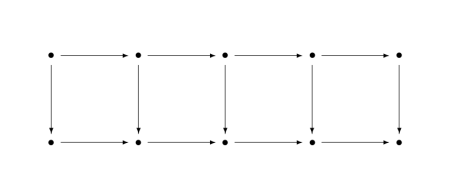Given assumptions about every arrow except the vertical arrow in the middle, the theorem concludes something about the middle arrow.## Nine lemma

The nine lemma, a.k.a. the 3×3 lemma, starts with a commutative diagram of the following shape.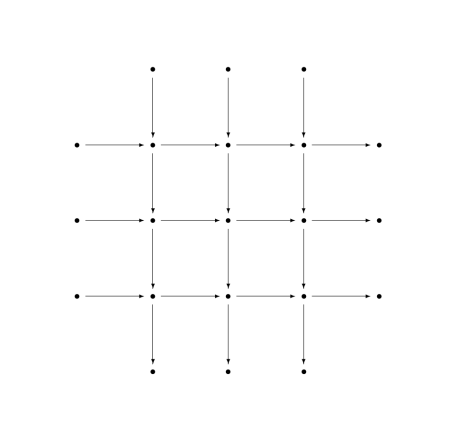It’s called the nine lemma because the attention is focused on the nine dots, the 3×3 grid of dots in the middle. All the objects around the perimeter, those outside the 3×3 grid, are 0.

There are three parts to the nine lemma. Each has hypotheses about all the columns and two of the rows, and concludes something about the row that was left out. So, for example, one part of the theorem has hypotheses about the three columns and the first two rows and concludes something about the last row.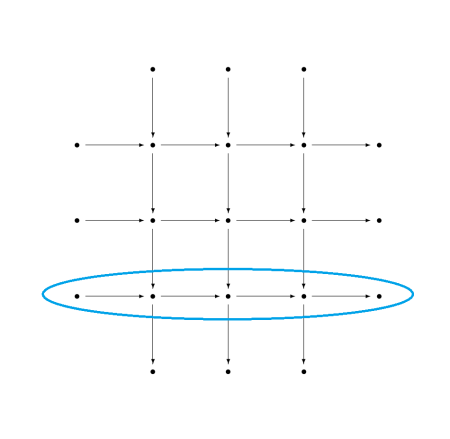## Missing details

Here I zoom in from 100,000 feet to 10,000 feet, giving some of the missing details but not all. For full details, you could look up the theorems on Wikipedia or on nLab.

As promised at the beginning, this was a ridiculously high-level view of these theorems. The purpose was to point out the core of each theorem and making it possible to compare the three theorems at the highest level.

When I said a theorem makes a hypothesis about a row or a column, the hypothesis is that the row forms an exact sequence. The conclusions in the nine lemma are that a row is an exact sequence.

When I alluded to hypotheses about vertical arrows, these hypotheses are essentially saying that a function is one-to-one (injective), onto (surjective), or one-to-one and onto (bijective). More precisely, they are assumptions that a morphism is mono, epi, or iso. More on this terminology here. The conclusion of the four lemma is that an arrow is either epi or mono. The conclusion of the five lemma is that an arrow is iso.

# 3D Go with identities

Let’s play a game that’s something like Go in three dimensions. Our game is played on the lattice of points in 3D that have integer coordinates. Someone places stones on two lattice points, and your job is to create a path connecting the two stones by adding stones to neighboring locations.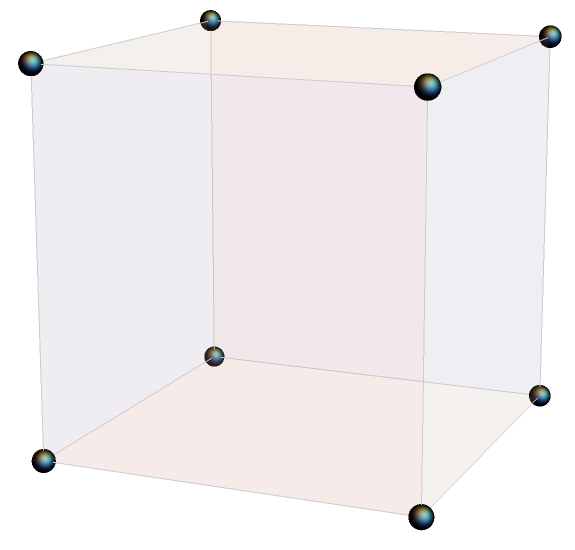## Game cube

We don’t really have a game “board” since we’re working in three dimensions. We have a game scaffold or game cube. And we can’t just put our stones down. We have to imagine the stones has having hooks that we can use to hang them where pieces of scaffolding come together.

## Basic moves

Now for the rules. Our game is based on identities for a function with depending on three parameters: a, b, and c. A location in our game cube is a point with integer coordinates (i, j, k) corresponding to function parameters

(a+i, b+j, c+k).

If there is a stone at (i, j, k) then we are allowed to place stones at neighboring locations if there is a function identity corresponding to these points. The function identities are the “contiguous relations” for hypergeometric functions. You don’t need to know anything about hypergeometric functions to play this game. We’re going to leave out a lot of detail.

Our rule book for identities will be Handbook of Mathematical Function by Abramowitz and Stegun, widely known as A&S. You can find a copy online here.

For example, equation 15.2.10 from A&S relates

F(a, b; c; z)

to

F(a-1, b; c; z)

and

F(a+1, b; c; z).

So if we have a stone at (i, j, k) we can add stones at (i-1, j, k) and (i+1, j, k). Or we could add stones at (i+1, j, k) and (i+2, j, k). You can turn one stone into a row of three stones along the first axis overlapping the original stone.

Equation 15.2.11 lets you do something analogous for incrementing the 2nd coordinate, and Equation 15.2.12 lets you increment the 3rd coordinate. So you could replace a stone with a row of three stones along the second or third axes as well.

This shows that it is always possible to create a path between two lattice points. If you can hop between stones a distance 1 apart, you can create a path that will let you hop between any two points.

There are other possible steps. For example, equation 15.2.25 has the form

F(a, b; c; z) + … F(a, b-1; c; z)  + … F(a, b; c+1; z) = 0

I’ve left out the coefficients above, denoting them by ellipses. (More on that below.) This identity says you don’t just have to place stones parallel to an axis. For example, you can add a stone one step south and another one step vertically in the same move.

The previous section showed that it’s always possible to create a path between two lattice points. This section implies the finding the shortest path between two points might be a little more interesting.

## Applications

There are practical applications to the game described in this post. One reason hypergeometric functions are so useful is that they satisfy a huge number of identities. There are libraries of hypergeometric identities, and there are still new identities to discover and new patterns to discover for organizing the identities.

The moves in the game above can be used to speed up software. Hypergeometric functions are expensive to evaluate, and so it saves time to compute a new function in terms of previously computed functions rather than having to compute it from scratch.

I used this trick to speed up clinical trial simulations. Consecutive steps in the simulation required evaluating contiguous hypergeometric functions, so I could compute one or two expensive functions at the beginning of the simulation and bootstrap them to get the rest of the values I needed.

## Coefficients

I’d like to close by saying something about the coefficients in these identities. The coefficients are complicated and don’t have obvious patterns, and so they distract from the underlying structure discussed here. You could stare at a list of identities for a while before realizing that apart from the coefficients the underlying relationships are simple.

All the coefficients in the identities cited above are polynomials in the variables a, b, c, and z. All have degree at most 2, and so they can be computed quickly.

The identities discussed here are often called linear relations. The relations are linear in the hypergeometric functions, but the coefficients are not linear.

# Area of spherical triangle

A few days ago I wrote about how you could calculate the area of a spherical triangle by measuring the angles at its vertices. As was pointed out in the comments, there’s a much more direct way to find the area.

Folke Eriksson gives the following formula for area in . If a, b, and c are three unit vectors, the spherical triangle formed by their vertices has area E whereLet’s try the formula out with an example and compare the area to that of a flat triangle. We’ll pick three cities as our three points, assuming the earth is a unit sphere.

Let a = Austin, Texas, b = Boston, Massachusetts, and c = Calgary, Alberta. The spherical coordinates of each point will be (1, θ, φ) where θ is longitude and φ is π/2 minus latitude.

    from numpy import *

def latlong_to_cartesian(latlong):
phi   = deg2rad( 90 - latlong )

x = sin(theta) * cos(phi)
y = sin(theta) * sin(phi)
z = cos(theta)
return array([x,y,z])

def spherical_area(a, b, c):
t = abs( inner(a, cross(b, c) ) )
t /= 1 + inner(a,b) + inner(b,c) + inner(a,c)
return 2*arctan(t)

def flat_area(a, b, c):
x = cross(a - b, c - b)
return 0.5*linalg.norm(x)

# Latitude and longitude
austin  = (30.2672,  97.7431)
boston  = (42.3584,  71.0598)
calgary = (51.0501, 114.0853)

a = latlong_to_cartesian(austin)
b = latlong_to_cartesian(boston)
c = latlong_to_cartesian(calgary)

round = spherical_area(a, b, c)
flat  = flat_area(a, b, c)
print(round, flat, round/flat)


This shows that our spherical triangle has area 0.1154 and the corresponding Euclidean triangle slicing through the earth would have area 0.1093, the former being 5.57% bigger.

Let’s repeat our exercise with a smaller triangle. We’ll replace Boston and Calgary with Texas cities Boerne and Carrollton.

    boerne  = (29.7947, 98.7320)
carrollton = (32.9746, 96.8899)


Now we get area 0.000345 in both cases. The triangle is so small relative to the size of the earth that the effect of the earth’s curvature is negligible.

In fact the ratio of the two computed areas is 0.9999, i.e. the spherical triangle has slightly less area. The triangle is so flat that numerical error has a bigger effect than the curvature of the earth.

How could we change our code to get more accurate results for small triangles? That would be an interesting problem. Maybe I’ll look into that in another post.

 “On the measure of solid angles”, F. Eriksson, Math. Mag. 63 (1990) 184–187.